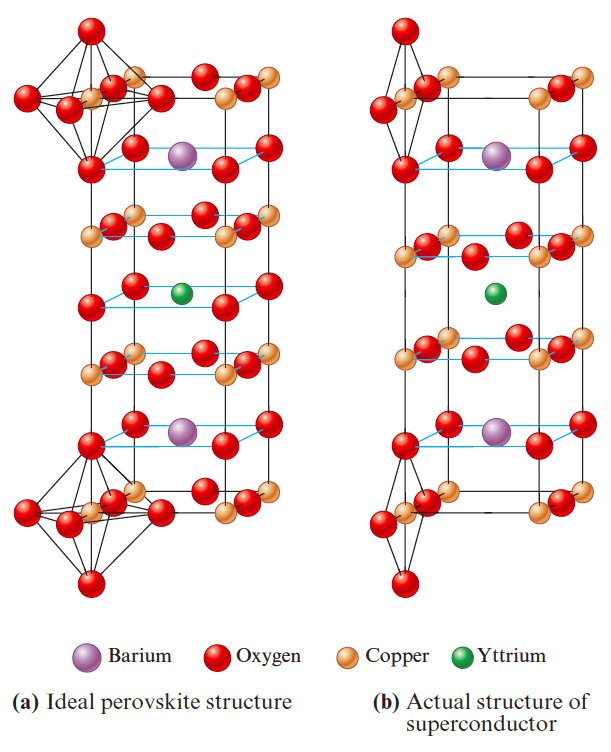# Problem: Materials containing the elements Y, Ba, Cu, and O that are superconductors (electrical resistance equals zero) at temperatures above that of liquid nitrogen were recently discovered. The structures of these materials are based on the perovskite structure. Were they to have the ideal perovskite structure, the superconductor would have the structure shown in part (a) of the following figure.What is the formula of this ideal perovskite material?

###### FREE Expert Solution
92% (176 ratings)
###### Problem Details

Materials containing the elements Y, Ba, Cu, and O that are superconductors (electrical resistance equals zero) at temperatures above that of liquid nitrogen were recently discovered. The structures of these materials are based on the perovskite structure. Were they to have the ideal perovskite structure, the superconductor would have the structure shown in part (a) of the following figure.What is the formula of this ideal perovskite material?